# Spline interpolation

Jump to: navigation, search

Interpolation by means of splines (cf. Spline), that is, the construction of an interpolation spline taking given valuesat prescribed points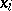,. Interpolation splines usually satisfy further conditions at the end points. E.g., for the cubic spline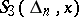, where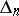is the partition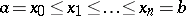, which, on, consists of piecewise-cubic polynomials and has a continuous second-order derivative, one requires thatand, in addition, one condition at each end point (e.g.,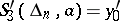and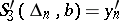, or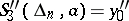and). If the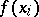are the values of a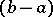-periodic function, then one requires the spline to be-periodic also. For polynomial splines of degree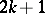, the number of extra conditions at each end pointoris increased by. For interpolation splines of degree, the knots of the spline (the points of discontinuity of the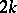-th derivative) are usually chosen halfway between the points, and a furtherconditions are assigned atand.

Spline interpolation has some advantages when compared to polynomial interpolation. E.g., there are sequences of partitions: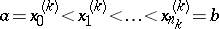and interpolation splines for which the interpolation process converges for any continuous function, provided that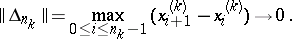Many processes of spline interpolation give the same order of approximation as the best approximation. Moreover, spline interpolation of some classes of differentiable functions has the property that the error does not exceed the width of the corresponding class. Spline interpolation can be used to solve certain variational problems. E.g., under sufficiently general additional conditions atand, interpolation splines satisfy the relation: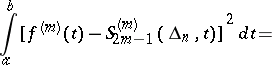(1)This implies the existence and uniqueness of interpolation splines of odd degree, and also the simplest results on convergence: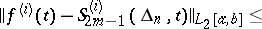(2a)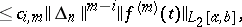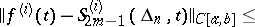(2b)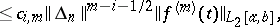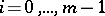, where the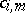depend only onand, and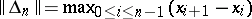. For some classes of differentiable functions, the sequence of interpolation splines converges to the function to be interpolated on any sequence of partitions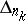for which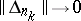(this occurs in case (2a)–(2b)).

In addition to polynomial interpolation splines, one can also use splines of a more general form (-splines or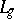-splines). For many of these, results analogous to (1) and (2a)–(2b) also hold. For splines with defect greater than 1 one usually carries out interpolation with multiple knots.

See also Spline approximation.

For references see Spline.